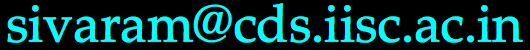# Constructive Approximation Theory, January-April 2017

When Tuesday and Thursdays morning from 8:30 AM to 10:00 AM
Where Department of Computational & Data Sciences, SERC building, Room 202, Seminar Hall

### Instructor

Sivaram Ambikasaran,Office: Department of Computational & Data Sciences, SERC building, Room 205.
Office hours: Tuesday 3:00 PM-5:00 PM or by appointment.

### Syllabus

The course will focus on constructive approximation of real valued functions by simpler function and numerical quadrature. We will discuss the mathematical theory and computational techniques of such approximations. Error bounds and convergence of approximations will be discussed. A substantial part of the course will be dedicated to polynomial approximations and quadratures. All the course material (error bounds, convergence, Weierstrass approximation theorem, optimal interpolant, Ramez algorithm) will be discussed and illustrated computationally. The emphasis of the course is on computational assignments, which will be due weekly. The highlight of the course would be how approximations lend themselves in constructing highly accurate fast algorithms. Topics: Approximation by Algebraic Polynomials, Weierstrass Theorem, Muentz-Szasz theorem, Orthogonal polynomials, Optimal interpolation nodes, Optimal interpolant, Lebesgue constants, Fourier series, Gibbs phenomenon, Potential theory and approximation, Spectral methods, Pade approximation, Clenshaw-Curtis and Gaussian quadrature, fast low rank construction of kernel matrices and other fast matrix computations.

### Textbooks

• Approximation Theory and Approximation Practice, by Lloyd N. Trefethen; The book is available here.
• Interpolation and Approximation by Polynomials, by George M. Phillips
• Chebyshev and Fourier spectral methods, by John P. Boyd

 Evaluation Homework Quiz Project Points 60 20 20

### Homework

There will be a total of 12 homework (each worth 5 points) due roughly weekly. Homework will be posted on this website and will be due on Wednesdays before 5 PM. Late homework will not be accepted. The homework will involve fair amount of programming exercises. Students are strongly encouraged to typeset their solutions using LaTeX/TeX (10% bonus points for submitting in LaTeX/TeX). To save trees, the students need to send their homework through email towith the subject reading CAT_2017_HW_#_firstname, where # needs to be replaced with the homework number (between 1 and 12) and firstname is to be replaced with your first name in lower case. Details on how to submit the computing part of the homework will be elaborated in the homework itself. No collaboration is allowed for homework.

The grader will expect you to express your ideas clearly, legibly, and completely, often requiring complete English sentences rather than merely just a long string of equations or unconnected mathematical expressions. This means you could lose points for poorly written proofs or answers. Clear exposition is a crucial ingredient of mathematical communication. Clarity of thought and presentation is more important in mathematics & sciences than any other field. The only way to master exposition is by repeated practicing.

#### $$\LaTeX$$

If you don't have $$\LaTeX$$ on your system, try any of the following online ones.
• https://www.sharelatex.com/
• https://www.overleaf.com/

### Computational requirement

Each homework will have couple of computational exercises. Students must be comfortable with programming and are expected to have working knowledge in C++ & MATLAB. If not, they should be able to learn and immediately pick it up.

#### C++ compilers

For C++ compilers, try any of the following (there any many other options if you google for "online compiler"). Pick your favorite one.
• http://www.tutorialspoint.com/compile_cpp11_online.php
• https://www.codechef.com/ide

### Quiz

There will be a short (3-5 minutes) quiz in almost every class.

### Project

This could be a work arising out a published article or from material/discussions in class. The project is due on April 12th. Below are some articles for suggestion.

### Calendar

Below is a calendar, which will be updated as we make progress in the course.
 Week Tuesday Thursday Homework Jan 9-13 Introduction, Polynomial interpolation for a wide range of functions, Runge phenomenon Fundamental theorem of polynomial interpolation, Started looking at Chebyshev and Legendre nodes Homework 1: Due on Jan 18 Jan 16-20 No class Orthogonal polynomials Homework 2: Due on Jan 25 Jan 23-27 Legendre polynomials Republic day Homework 3: Due on Feb 1 Jan 30-Feb 3 Chebyshev polynomials Weierstrass approximation theorem Homework 4: Due on Feb 8 Feb 6-10 Best approximation Homework 5: Due on Feb 15 Feb 13-17 Homework 6: Due on Feb 22 Feb 20-24 Feb 27-Mar 3 Homework 7: Due on Mar 8 Mar 6-Mar 10 Homework 8: Due on Mar 13 Mar 13-17 Homework 9: Due on Mar 17 Mar 20-24 Homework 10: Due on April 12 Mar 27-31 Homework 11: Due on April 19 Apr 3-7 Project due on Apr 12 and final report due on April 19